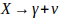# Not yet finished, have gotten permission to extend the final deadline to 30/06/20

## I still have left to finish the plots and the code to do all the calculations, but it is under progress, and i will add it as soon as it is complete. Other than than i have to add the equations to the text, and finish the conclusion. I will be done with this before the 30. of june. I will also add my references.

Unstable Dark Matter in the Milky Way

This is my project for the course PHYS 291 - Data Handling in Physics, by Nora Grebstad

Introduction

This project looks at the decay of a hypothetical dark matter particle to one photon and one neutrino. Assuming the resting energy of the particle is 100 GeV, this is used to find the energy of the gamma-photon emitted during decay.

In this project, the Navarro-Frenk-White profile for dark matter mass distribution is used. From this distribution it is possible to find the amount of dark matter particles that are enclosed by a halo, knowing the radius of the halo and using the earlier assumed mass of the particle.

A plot showing the photon flux of gamma radiation for a range of photon-energies.

By using a line-of-sight integral to find the photon flux by inserting an assumed lifetime of dark matter, and comparing it to the observations, one can find the lowest possible lifetime which are not in conflict with the observations. The resulting lower limit for the lifetime will be discussed in the conclusion. It will also be discussed whether the Navarro-Frenk-White profile for dark matter is a fitted model in this project.

Dark Matter

Back in 1993, Fritz Zwicky Comahopen examined a cluster of galaxies consisting of more than a thousand galaxies over 300 lightyears away . He discovered that the galaxies were moving too fast compared to the mass calculated from the observed intensity of the light. He concluded that for the galaxies not to be torn apart, the cause of these observations had to be a mass not interacting with light. He named this mysterious mass dark matter . Since then many theories about what dark matter is has occurred, and it has become a leading paradigm to explain several phenomenons in our universe.

Dark matter is believed to take up about 25 percent of the universe we have today, with only 5 percent made up by the particles in the standard model, and the remaining 70 percent by dark energy . Since the particles are "dark", and does not interact with electromagnetic waves, they must be observed in a different way than other particles that we know. The dark matter in the Milky Way is believed to be concentrated in the centre.

One method used as an attempt to detect dark matter is to look for gamma-rays, antiprotons or positrons created by decay of a dark matter particle.

Decay

Assume a dark matter particle X with mass mx = 100 GeV/c^2. Also assume this particle decay into a photon and a neutrino:

Since the neutrino is very hard to measure, it is the photon that is studied. If we are in a reference system where the X-particle is at rest, we can use the laws of momentum and energy conservation

Because of the negligible mass of the neutrino, we can assume it to have zero mass. The two formulas above leads to the energy of the photon

Navarro-Frenk-White halo profile

The profile is a developed theoretical profile for the mass distribution of dark matter in the halo of matter surrounding the Milky Way. The distribution is given by the formula

where po = 0.33 and rc = 20 kpc.

Line-of-Sight Integral

To find the photon flux from the dark matter by the earth’s surface, one can derive a line-of-sight integral over the halo profile, which is given by the formula

where tau is the lifetime of the particle, which is not known. If rj is the distance from the centre of the galaxy to the surface of the earth, one can use the formula

This figure shows the angle theta

to get a new formula for the photon flux, where theta is the angle between the line intersecting the earth and the centre of the Milky Way, and the line along which the integral is taken

Method

This figure shows the observed energy flux at the earth’s surface for different photon energies. Where the vertical line intersects with the measurements, are the values I want to study in this project, which comes from the earlier assumption of the mass of the dark matter particle.

I want to use the line-of-sight integral to find a value of the lifetime which is not in conflict with the observations in the figure. In other words, the calculated result has to lie within the uncertainty range of the point on the figure.

To find a fitting value for the photon flux, I insert different values of tau, starting with tau = 10^15. I take the integral with program for small angles, because most of the dark matter has to be concentrated in the centre of the galaxy. I then calculate the mean value of the photon flux values for all the angles with program, and compare the result to the observations in the figure. I find that the best value for the lifetime of the particle is tau = ?.

The photon flux values for each angle for this value of the lifetime is

(table)

This plot shows how the photon flux varies with the angle

(plot)

Conclusion